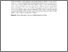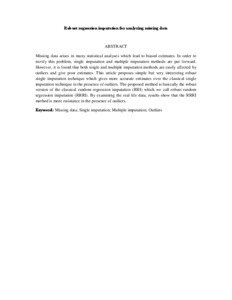# Robust regression imputation for analyzing missing data

## Citation

Rana, Md. Sohel and John, Ahamefule Happy and Midi, Habshah (2012) Robust regression imputation for analyzing missing data. In: 2012 International Conference on Statistics in Science, Business and Engineering (ICSSBE 2012), 10-12 Sept. 2012, Langkawi, Kedah. .

## Abstract

Missing data arises in many statistical analyses which lead to biased estimates. In order to rectify this problem, single imputation and multiple imputation methods are put forward. However, it is found that both single and multiple imputation methods are easily affected by outliers and give poor estimates. This article proposes simple but very interesting robust single imputation technique which gives more accurate estimates over the classical single imputation technique in the presence of outliers. The proposed method is basically the robust version of the classical random regression imputation (RRI) which we call robust random regression imputation (RRRI). By examining the real life data, results show that the RRRI method is more resistance in the presence of outliers.Preview
Text (Abstract)
Robust regression imputation for analyzing missing data.pdfView Item